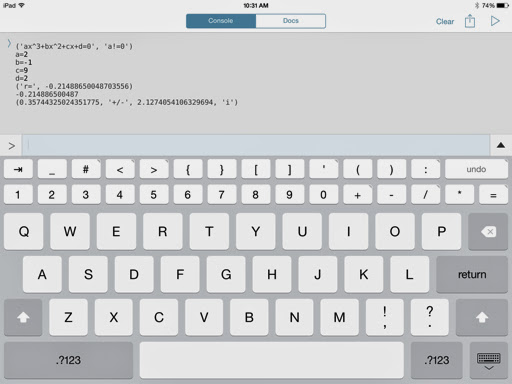## Thursday, July 31, 2014

### Roots of the Cubic Equation - Pythonista 2.7Roots of the Cubic Equation - Pythonista 2.7

Script:

# Cubic Equations: 2014-07-30 EWS
# need the root
import math
print('ax^3+bx^2+cx+d=0','a!=0')
a=float(input('a='))
b=float(input('b='))
c=float(input('c='))
d=float(input('d='))
# is a root 1?
test=a+b+c+d
if test==0:
r=1
if test!=0:
# first root by Newton's Method
xn=1
x1=xn-(a*xn**3+b*xn**2+c*xn+d)/(3*a*xn**2+2*b*xn+c)
while math.fabs(xn-x1)>1e-13:
xn=x1
x1=xn-(a*xn**3+b*xn**2+c*xn+d)/(3*a*xn**2+2*b*xn+c)
r=x1
print('r=',r)
# second and third roots
ap=a
bp=a*r+b
cp=a*r**2+b*r+c
disc=bp**2-4*ap*cp
if disc<0:
real=-bp/(2.*ap)
imag=math.sqrt(math.fabs(disc))/(2.*ap)
print(r)
print(real,'+/-',imag,'i')
else:
s=(-bp+math.sqrt(disc))/(2.*a)
t=(-bp-math.sqrt(disc))/(2.*a)
print(r)
print(s)
print(t)

Examples:

x^3 - 4*x^2 - 17*x + 60 = 0
a = 1, b = -4, c = -17, d = 60
Roots: 3, 4, 5

2*x^3 + x^2 - 2*x + 1 = 0
a = 2, b = 1, c = -2, d = 1
Roots (approximately): -1.43756, 0.46878 ± 0.35784i

This blog is property of Edward Shore. 2014

### Swiss Micros DM16L: Advanced Boolean and Factorial (up to 20)

Swiss Micros DM16L:   Advanced Boolean and Factorial (up to 20) Introduction The program listing, for the Swiss Micros DM16L and Hewlett Pac...